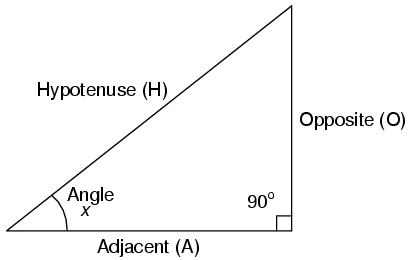# APPENDIX A: Right Triangle TrigonometryA right triangle is defined as having one angle precisely equal to 90o  (a right angle).

# Trigonometric Identities

$Sin x\:=\frac{O}{H}$

$Cos x\:=\frac{A}{H}$

$Tan x\:=\frac{O}{A}$

$Tan x\:=\frac{Sin x}{Cosx}$

$csc x\:=\frac{H}{O}$

$sec x\:=\frac{H}{A}$

$cot x\:=\frac{A}{O}$

$cot x\:=\frac{Cosx}{Sinx}$

H is the Hypotenuse, always being opposite the right angle. Relative to angle x, O is the Opposite and A is the Adjacent. “Arc” functions such as “arcsin”, “arccos”, and “arctan” are the complements of normal trigonometric functions. These functions return an angle for a ratio input. For example, if the tangent of 45o is equal to 1, then the “arctangent” (arctan) of 1 is 45o. “Arc” functions are useful for finding angles in a right triangle if the side lengths are known.

# The Pythagorean Theorem

$H^2 =A^2 +O^2$